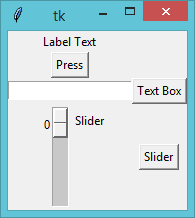## Pages

### Tkinter - Keyboard Input

In this example we have added two new fucntions and attached them to the events of a set key being pressed. This triggers set functions to be ran.
```import tkinter as tk

mywindow = tk.Tk()

def buttonPress():
print("Button Pressed!!")

def textBox():
print(textb.get())

def slideValue():
print (Slider.get())

#Label
label = tk.Label(mywindow, text="Label Text")
label.grid(row=0,column=1)

#Button
button = tk.Button(mywindow,text='Press',command=buttonPress)
button.grid(row=1,column=1)

#Textbox
textb = tk.Entry(mywindow,text="Entry")
textbutton = tk.Button(mywindow,text="Text Box",command=textBox)
textb.grid(row=2,column=1)
textbutton.grid(row=2,column=2)

#Slider
Slider = (tk.Scale(mywindow,label=('Slider'),variable=0))
SliderButton = (tk.Button(mywindow,text='Slider',command=slideValue))
Slider.grid(row=3,column=1)
SliderButton.grid(row=3,column=2)

def func_return(event):
print ("You hit return")
mywindow.bind("<Return>", func_return)

def func_w(event):
print ("You hit w")
mywindow.bind("<KeyPress-w>", func_w)

mywindow.mainloop()
```
This creates the same frame:But when return or "w" are pressed after each other, the follwoing is returned.
```You hit return
You hit w
You hit return
You hit w
You hit return
You hit w
You hit return
You hit w
You hit return
```

## Related Sections

Python - Learn the python from the basics up. This fast track example code course will get you creating powerful python programs in no time.
Tkinter - Learn the key features of the tkinter to allow you to create user interfaces for your python programs.